## Example Questions

### Example Question #41 : Arithmetic

A TV show lasts 30 minutes, what fraction of the show is left after 12 minutes have passed?

4/10

2/3

3/5

12/15

1/3

3/5

Explanation:

After watching 12 minutes of the show 18 remain. 18 is 60% of the total 30 minutes. As a fraction it can be expressed as 3/5.

### Example Question #50 : Proportion / Ratio / Rate

A bag contains red, orange, and yellow marbles only. The marbles occur in a ratio of 5 red marbles: 4 orange marbles: 1 yellow marble. If one-third of the red marbles, one-half of the orange marbles, and one-fourth of the yellow marbles are removed, then what fraction of the remaining marbles in the bag is red?

39/73

43/75

40/73

40/43

37/77

40/73

Explanation: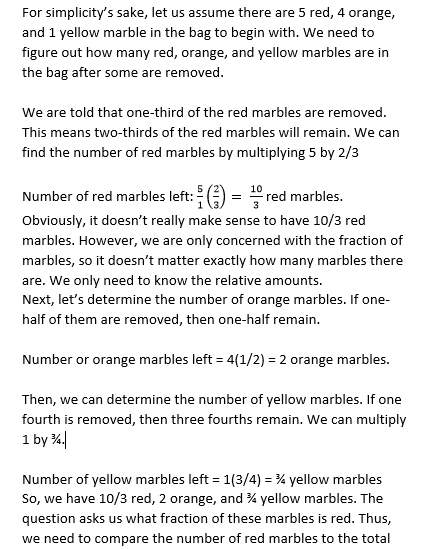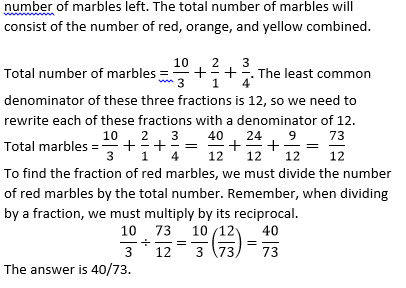### Example Question #1 : How To Find The Ratio Of A Fraction

Marty drove 40 mi/hr for 3 hours, then 60 mi/hr for 1 hour, and finally 70 mi/hr for the last 2 hours. What was Marty's average speed?

53 mi/hr

60 mi/hr

47 mi/hr

58 mi/hr

63 mi/hr

53 mi/hr

Explanation:

Marty's total driving time was 3 + 1 + 2 = 6 hours. He drove 40 mi/hr for 3 hours, or 3/6 = 1/2 of the time. He drove 60 mi/hr for 1 hour, or 1/6 of the drive. Lastly, he drove 70 mi/hr for 2 hours, or 2/6 = 1/3 of the drive.

To find the average speed, we need to multiply the speeds with their corresponding weights and add them up.

Average = 1/2 * 40 + 1/6 * 60 + 1/3 * 70 = 53.33... ≈ 53 mi/hr

### Example Question #1 : How To Find The Ratio Of A Fraction

A pie is made up ofcrust,apples, andsugar, and the rest is jelly. What is the ratio of crust to jelly?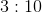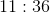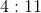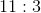Explanation:

A pie is made up ofcrust,apples,sugar, and the rest is jelly. What is the ratio of crust to jelly?

To compute this ratio, you must first ascertain how much of the pie is jelly. This is: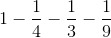Begin by using the common denominator: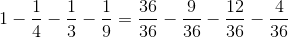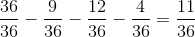So, the ratio of crust to jelly is: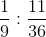This can be written as the fraction: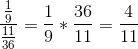, or### Example Question #1 : How To Find The Ratio Of A Fraction

In a solution,of the fluid is water,is wine, and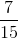is lemon juice. What is the ratio of lemon juice to water?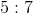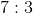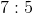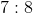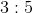Explanation:

This problem is really an easy fraction division. You should first divide the lemon juice amount by the water amount: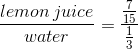Remember, to divide fractions, you multiply by the reciprocal: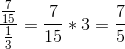This is the same as saying:### Example Question #5 : How To Find The Ratio Of A Fraction

If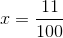and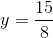, what is the ratio ofto?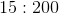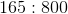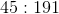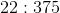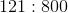Explanation:

To find a ratio like this, you simply need to make the fraction that represents the division of the two values by each other. Therefore, we have: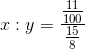Recall that division of fractions requires you to multiply by the reciprocal: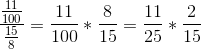which is the same as: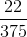This is the same as the ratio: